• 仿射变换含义 仿射变换是指在几何中，一个向量空间（vector space）进行一次线性变换（linear transformation）并拼上一个平移（Translation ）。所以，本质上仿射变换针对的是某一向量空间（当然该空间中的任一向...
仿射变换含义

仿射变换是指在几何中，一个向量空间（vector space）进行一次线性变换（linear transformation）并拼上一个平移（Translation ）。所以，本质上仿射变换针对的是某一向量空间（当然该空间中的任一向量）。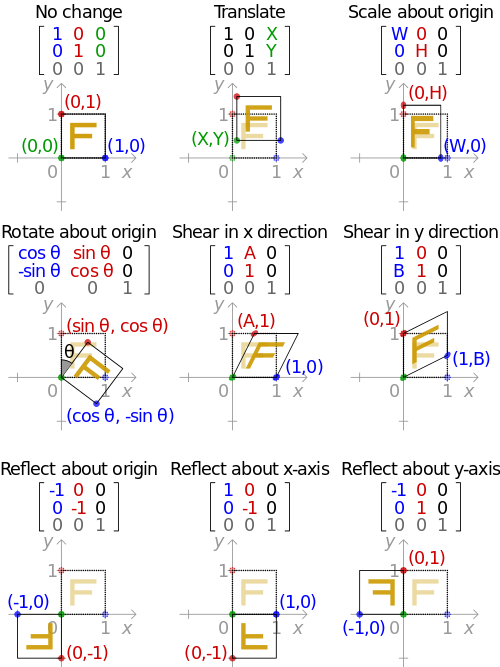其矩阵表达形式（matrix formal）为：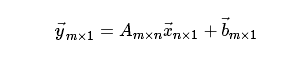A控制旋转与缩放，b控制平移：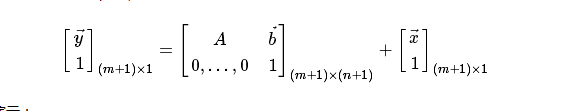我们使用Python语言对之进行演示：
import numpy as np
x = np.array((1, 2, 3))
b = np.ones(2)
A = np.random.rand(2, 3)
y1 = np.dot(A, x) + b
#  array([ 4.17327185,  4.0755495 ])

A_aug = np.hstack((A, b.reshape((-1, 1))))
A_aug = np.vstack((A_aug, np.hstack(np.zeros(3), 1).reshape((1, -1))))
y2 = np.dot(A_aug, np.hstack((x, 1)).reshape((-1, 1)))
# array([[ 4.17327185],
# [ 4.0755495 ],
# [ 1.        ]])

# 上述构造的方式仍稍显繁琐，这里再提供一种方式
A_aug = np.zeros((A.shape+1, A.shape+1))
A_aug[-1, -1] = 1
A_aug[:-1, :-1] = A
A_aug[:-1, -1] = b
np.dot(A_aug, np.hstack((x, 1)).reshape((-1, 1)))
展开全文python
• 仿射变换过程，(x,y)表示原图像中的坐标，(x’,y’)表示目标图像的坐标 ↑ 三. 仿射变换——图像平移 算法： 仿射变换—图像平移算法，其中tx为在横轴上移动的距离，ty为在纵轴上移动的距离 ↑ 四. python实现...
• 什么是仿射变换？3. 定义4. 常用的转换矩阵5.实际应用5.1 栗子15.2 栗子2 1. 引用 opencv学习(三十五)之仿射变换warpAffine 2. 什么是仿射变换？ 3. 定义 向量空间中进行一次线性变换(乘以一个矩阵A)再加上一个平...


文章目录
1. 引用2. 什么是仿射变换？3. 定义4. 常用的转换矩阵5.实际应用5.1 栗子15.2 栗子2

1. 引用
opencv学习(三十五)之仿射变换warpAffine
2. 什么是仿射变换？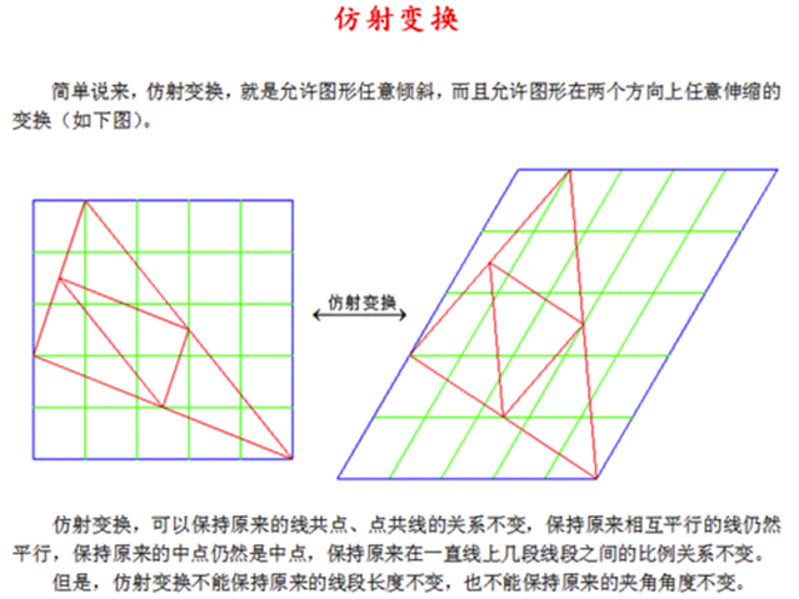3. 定义
向量空间中进行一次线性变换(乘以一个矩阵A)再加上一个平移(加上一个向量B)。 现定义参数A，B和AB的组合M(固定尺寸2*3)：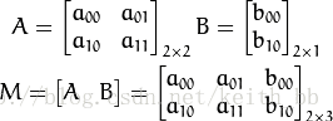定义自变量(起始量)：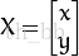则X → T 的仿射变换过程可以表达式为：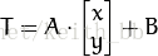或者：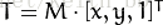得到结果T：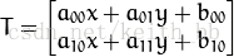其实可以看出，矩阵M中a决定缩放旋转等性质，b决定平移性质。若a为单位矩阵，则M实际是表示平移b的效果： 仿射变换(Affine Transformation)
4. 常用的转换矩阵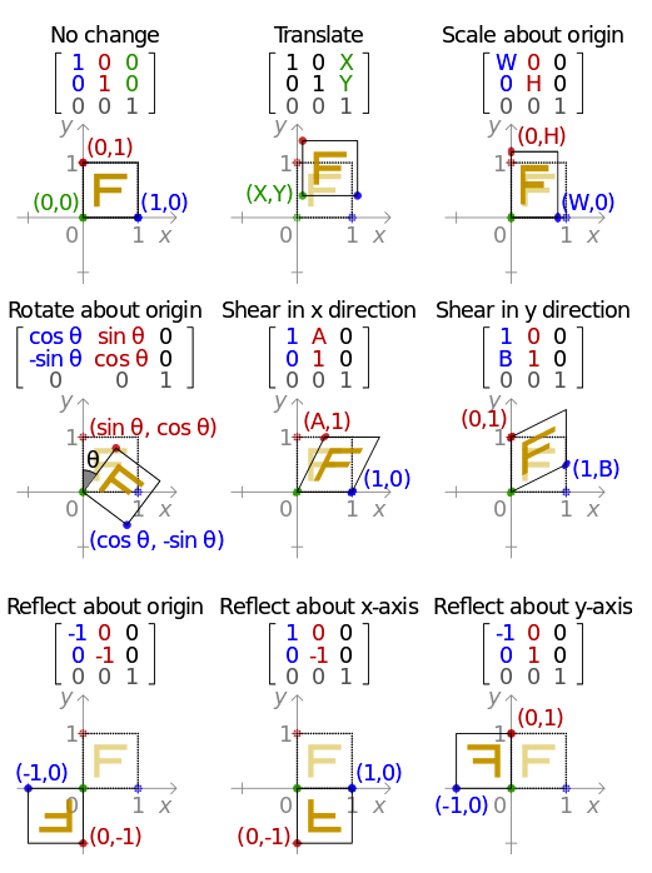5.实际应用
我们知道仿射变换一句话概括：原始图像IMG1在矩阵M的变换下，得到结果图像IMG2。 那么实际的应用就分两种情况：
知自变量和因变量X和T，求参数M(即A和B)【求关系–M代表IMG1和IMG2的关系】知自变量X和参数M，求结果T 【求结果–知道IMG1和矩阵M，就结果图】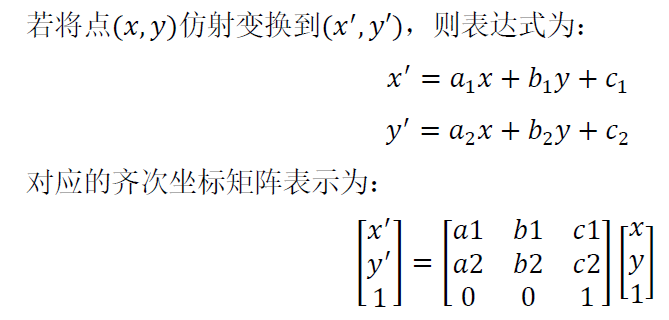由上式可见，完成仿射变换的公式含有6个未知数，则需要6个公式来表示才可解，所以完成图像的仿射变换需要三个点的对应关系，从而解出6个未知数，通过仿射公式对全部图像像素点进行仿射变换，所以在实际应用中需要先知晓3对坐标点的位置，计算出映射关系的仿射矩阵𝑀𝑀，再对全部像素进行仿射变换运算得到仿射后的图，完成仿射变换。
5.1 栗子1
在openCV中： 比如将128*128的图像上移30，左移30，则：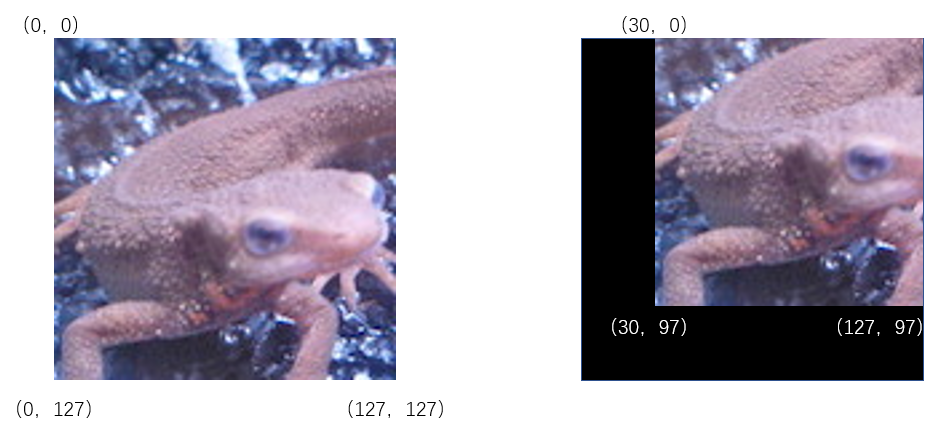我们已经得到有三对相应点
# 定义原图中三个点pts1
pts1=np.float32(([0,0],[0,127],[127,127]))

# 定义结果图中三个点pts2
pts2=np.float32(([30,0],[30,97],[127,97]))

# 利用这三对点，求出变换矩阵M
M=cv2.getAffineTransform(pts1,pts2)

# 再利用变换矩阵对原图所有像素点进行变换
# 并指定了输出图像的shape和原图shape一样
res=cv2.warpAffine(img,M,dsize=img.shape[0:2])

cv2.imshow("",res)
cv2.waitKey(0)5.2 栗子2
使用仿射变换，将图片在x方向上放大1.3倍，在y方向上缩小至原来的4/5。 对应的坐标如下：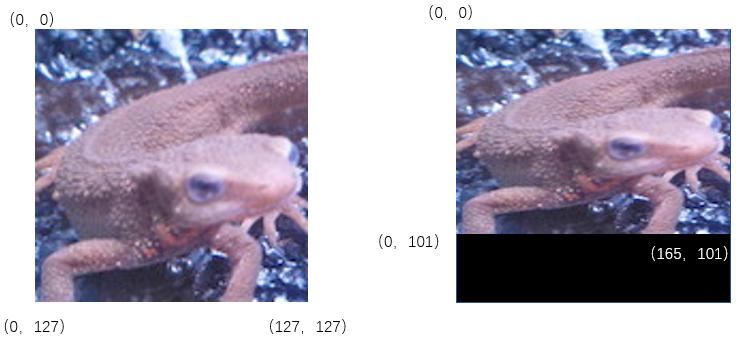代码如下：
import numpy as np
import cv2

pts1=np.float32(([0,0],[0,127],[127,127]))
pts2=np.float32(([0,0],[0,101],[165,101]))

M=cv2.getAffineTransform(pts1,pts2)
# 在定义输出图像尺寸时，从上图可以看出，有效图片在165*101部分
res=cv2.warpAffine(img,M,(165,101))

cv2.imshow("",res)
cv2.waitKey(0)如果在上面的结果之上，同时在x方向上向右平移30，在y方向上向上平移30呢？ 对应坐标为：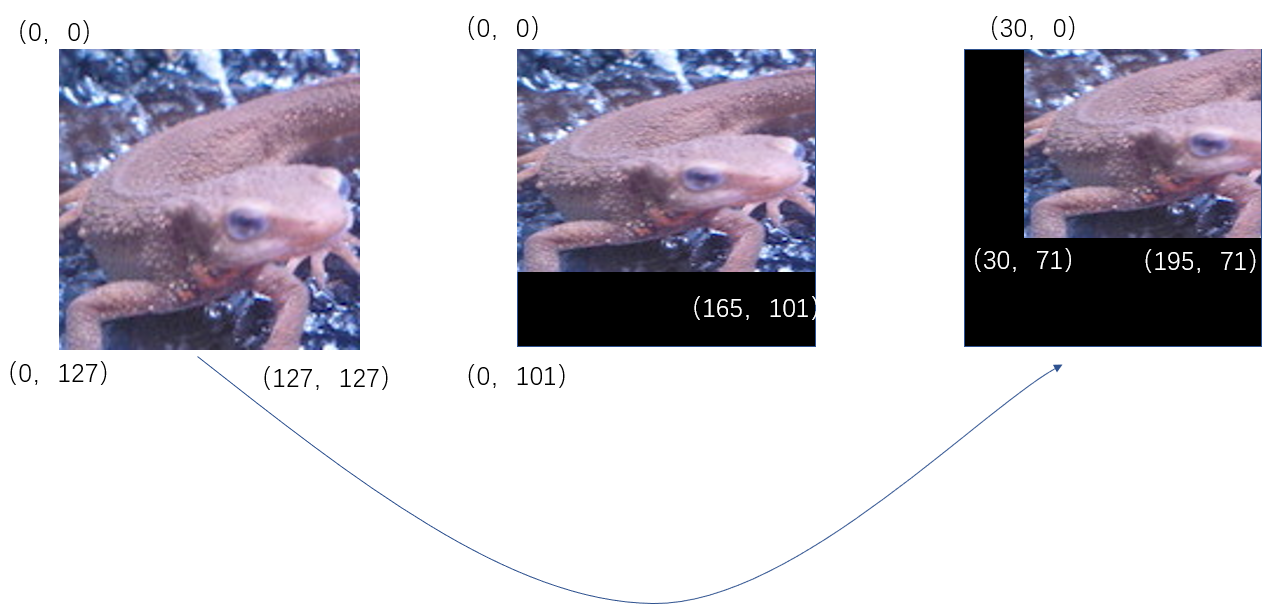如法炮制，代码：
import numpy as np
import cv2

pts1=np.float32(([0,0],[0,127],[127,127]))
pts2=np.float32(([30,0],[30,71],[195,71]))

M=cv2.getAffineTransform(pts1,pts2)
# 在定义输出图像尺寸时，从上图可以看出，有效图片在165*101部分
res=cv2.warpAffine(img,M,(165,101))

cv2.imshow("",res)
cv2.waitKey(0)展开全文• ## 一文搞懂仿射变换

千次阅读 多人点赞 2020-02-12 11:57:50
在图像处理中，我们经常需要对图像进行各种操作如平移、缩放、旋转、翻转等，有时候我们还需要将图像投影到另一个平面来对图像做一些矫正，然而这些操作都与仿射变换和透视变换有着莫大的联系。通过本篇文章，你能够...
导读
在图像处理中，我们经常需要对图像进行各种操作如平移、缩放、旋转、翻转等，这些其实都是图像的仿射变换。通过本篇文章，你能够知道它们的实现原理以及如何应用它们。
仿射变换
仿射变换也称仿射投影，是指几何中，对一个向量空间进行线性变换并接上一个平移，变换为另一个向量空间。所以，仿射变换其实也就是再讲如何来进行两个向量空间的变换 假设有一个向量空间

k

k

：

k

=

(

x

,

y

)

k=(x,y)

还有一个向量空间

j

j

：

j

=

(

x

′

,

y

′

)

j=(x',y')

如果我们想要将向量空间由

k

k

变为

j

j

，可以通过下面的公式进行变换

j

=

k

∗

w

+

b

j = k* w + b

将上式进行拆分可得

x

′

=

w

00

∗

x

+

w

01

∗

y

+

b

0

y

′

=

w

10

∗

x

+

w

11

∗

y

+

b

1

x'=w_{00}*x+w_{01}*y+b_0 \\ y'=w_{10}*x+w_{11}*y+b_1

我们再将上式转换为矩阵的乘法

[

x

′

y

′

]

=

[

w

00

w

01

b

0

w

10

w

11

b

1

]

[

x

y

1

]

=

M

[

x

y

1

]

(3)

\left[ \begin{matrix} x' \\ y' \\ \end{matrix} \right] \tag{3}= \left[ \begin{matrix} w_{00} & w_{01} & b_0 \\ w_{10} & w_{11} & b_1\\ \end{matrix} \right] \left[ \begin{matrix} x \\ y \\ 1 \end{matrix} \right]= M \left[ \begin{matrix} x\\ y\\ 1 \end{matrix} \right]

通过参数矩阵

M

M

就可以实现两个向量空间之间的转换，在进行仿射变换的时候我们也只需要一个矩阵

M

M

就可以实现平移、缩放、旋转和翻转变换。
接下来，会先介绍原理然后利用OpenCV来实现相应的例子，这里主要利用OpenCV的warpAffine函数来实现仿射变换
warpAffine函数参数：
src：输入的图像数组M：仿射变换矩阵dsize：变换后图像的大小flags：使用的插值算法borderValue：边界的填充值
图像平移
在平面坐标系有点

P

(

x

,

y

)

P(x,y)

和点

P

′

(

x

′

,

y

′

)

P'(x',y')

，如果我们想要将

P

P

点移动到

P

′

P'

通过下面的变换就可以实现

x

′

=

x

+

Δ

x

y

′

=

y

+

Δ

y

x'=x+\Delta x \\ y' = y + \Delta y

其中

Δ

x

\Delta x

和

Δ

y

\Delta y

就是x方向上和y方向上的偏移量，我们将其转换为矩阵的形式

[

x

′

y

′

]

=

[

1

0

Δ

x

0

1

Δ

y

]

[

x

y

1

]

=

M

[

x

y

1

]

\left[ \begin{matrix} x'\\ y'\\ \end{matrix} \right]= \left[ \begin{matrix} 1 & 0 & \Delta x\\ 0 & 1 & \Delta y\\ \end{matrix} \right] \left[ \begin{matrix} x\\ y\\ 1 \end{matrix} \right]= M\left[ \begin{matrix} x\\ y\\ 1 \end{matrix} \right]

上面的矩阵

M

M

就是仿射变换的平移参数，接下来我们利用OpenCV中的warpAffine函数来实现
import cv2
import numpy as np
import matplotlib.pyplot as plt

def show_compare_img(original_img,transform_img):
_,axes = plt.subplots(1,2)
#显示图像
axes.imshow(original_img)
axes.imshow(transform_img)
#设置子标题
axes.set_title("original image")
axes.set_title("warpAffine transform image")
plt.show()

def translation_img():
# 定义一个图像平移矩阵
# x向左平移(负数向左,正数向右)20个像素
# y向下平移(负数向上,正数向下)50个像素
M = np.array([[1, 0, -20], [0, 1, 50]], dtype=np.float)
# 读取需要平移的图像
# 将图片由BGR转为RGB
img = cv2.cvtColor(img, cv2.COLOR_BGR2RGB)
# 定义平移后图像的大小,保持和原图大小一致
dsize = img.shape[:2][::-1]
# 便于大家观察这里采用白色来填充边界
translation_img = cv2.warpAffine(img, M, dsize, borderValue=(255, 255, 255))
# 显示图像
show_compare_img(img, translation_img)

translation_img()图像翻转
有时候我们我们需要对图像进行水平翻转、垂直翻转、镜像翻转(同时进行水平和垂直翻转)，想要实现这个功能并不难，我们可以通过opencv内置的flip方法很容易实现，还可以通过numpy的索引来实现，这里我们主要介绍通过仿射变换矩阵来实现这个功能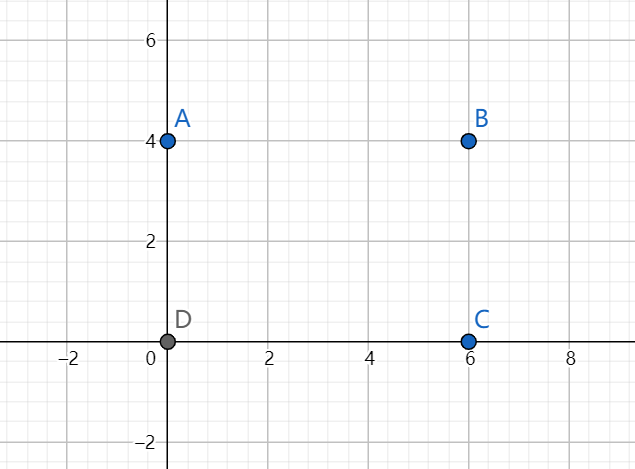上图中的

A

、

B

、

C

、

D

A、B、C、D

表示图像的四个顶点，如果我们需要对图像进行水平翻转，那么我们就需要将

A

A

点和

B

B

点进行交换，

C

C

点和

D

D

点进行交换，沿着x轴的中线进行对称交换位置，通过下面的式子可以实现水平翻转

x

′

=

−

x

+

w

\begin{aligned} x' = -x + w \end{aligned}

上式中的

w

w

表示图像的宽，同理可得垂直翻转的实现公式

y

′

=

−

y

+

h

\begin{aligned} y' = -y + h \end{aligned}

上式中的

h

h

表示的是图像的高
变换矩阵翻转图像 图像翻转的变换矩阵

水

平

翻

转

的

变

换

矩

阵

：

M

=

[

−

1

0

w

0

1

0

]

垂

直

翻

转

的

变

换

矩

阵

：

M

=

[

1

0

0

0

−

1

h

]

镜

像

变

换

的

矩

阵

：

M

=

[

−

1

0

w

0

−

1

h

]

\begin{aligned} 水平翻转的变换矩阵：\\ M=\left[ \begin{matrix} -1 & 0 & w\\ 0 & 1 & 0\\ \end{matrix} \right]\\ 垂直翻转的变换矩阵：\\ M=\left[ \begin{matrix} 1 & 0 & 0\\ 0 & -1 & h\\ \end{matrix} \right]\\ 镜像变换的矩阵：\\ M=\left[ \begin{matrix} -1 & 0 & w\\ 0 & -1 & h\\ \end{matrix} \right] \end{aligned}

def flip_img(horizontal_flip,vertical_flip,img):
#获取输入图片的宽和高
height,width = img.shape[:2]
#初始化变换矩阵
M = np.array([[0, 0, 0], [0, 0, 0]], dtype=np.float)
#水平翻转
if horizontal_flip:
M = [-1,0,width]
#垂直翻转
if vertical_flip:
M = [0,-1,height]
# 将图片由BGR转为RGB
img = cv2.cvtColor(img, cv2.COLOR_BGR2RGB)
# 定义缩放后图片的大小
img_flip = cv2.warpAffine(img, M, (width,height))
# 显示图像
show_compare_img(img, img_flip)

flip_img(True,True,img)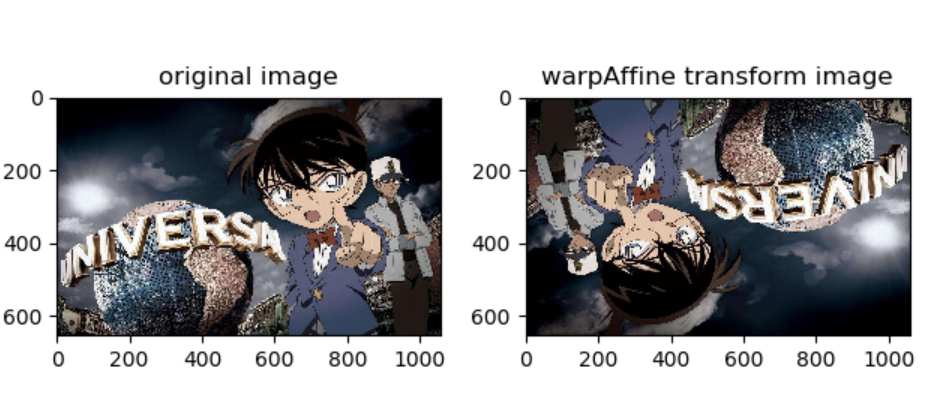OpenCV的flip函数翻转图像
flip函数参数：
src：输入的图像数组flipCode：图像翻转参数，1表示水平翻转，0表示垂直翻转，-1表示镜像翻转
img = cv2.imread("test.jpg")
#水平翻转
horizontal_flip_img = cv2.flip(img,1)
#垂直翻转
vertical_flip_img = cv2.flip(img,0)
#镜像翻转
mirror_flip_img = cv2.flip(img,-1)

numpy的索引翻转图像
img = cv2.imread("test.jpg")
#水平翻转
horizontal_flip_img = img[:,::-1]
#垂直翻转
vertical_flip_img = img[::-1]
#镜像翻转
mirror_flip_img = img[::-1,::-1]

图像缩放
如果我们想要对坐标系的

P

P

点进行缩放操作，通过下面的公式就可以实现

x

′

=

f

x

∗

x

y

′

=

f

y

∗

y

x'=f_x * x\\ y'=f_y * y

通过，在

x

x

和

y

y

前面添加一个缩放系数即可，同样我们将其转换为矩阵形式

[

x

′

y

′

]

=

[

f

x

0

0

0

f

y

0

]

[

x

y

1

]

=

M

[

x

y

1

]

\left[ \begin{matrix} x'\\ y'\\ \end{matrix} \right]= \left[ \begin{matrix} f_x & 0 & 0\\ 0 & f_y & 0\\ \end{matrix} \right] \left[ \begin{matrix} x\\ y\\ 1 \end{matrix} \right]= M\left[ \begin{matrix} x\\ y\\ 1 \end{matrix} \right]

通过上面的矩阵

M

M

我们就可以实现对图片的缩放
def scale_img():
#定义宽缩放的倍数
fx = 0.5
#定义高缩放的倍数
fy = 2
#定义一个图像缩放矩阵
M = np.array([[fx,0,0],[0,fy,0]],dtype=np.float)
#读取图像
#获取图片的宽和高
height,width = img.shape[:2]
#将图片由BGR转为RGB
img = cv2.cvtColor(img,cv2.COLOR_BGR2RGB)
#定义缩放后图片的大小
scale_img = cv2.warpAffine(img,M,(int(width*fx),int(height*fy)))
#显示图像
show_compare_img(img,scale_img)

scale_img()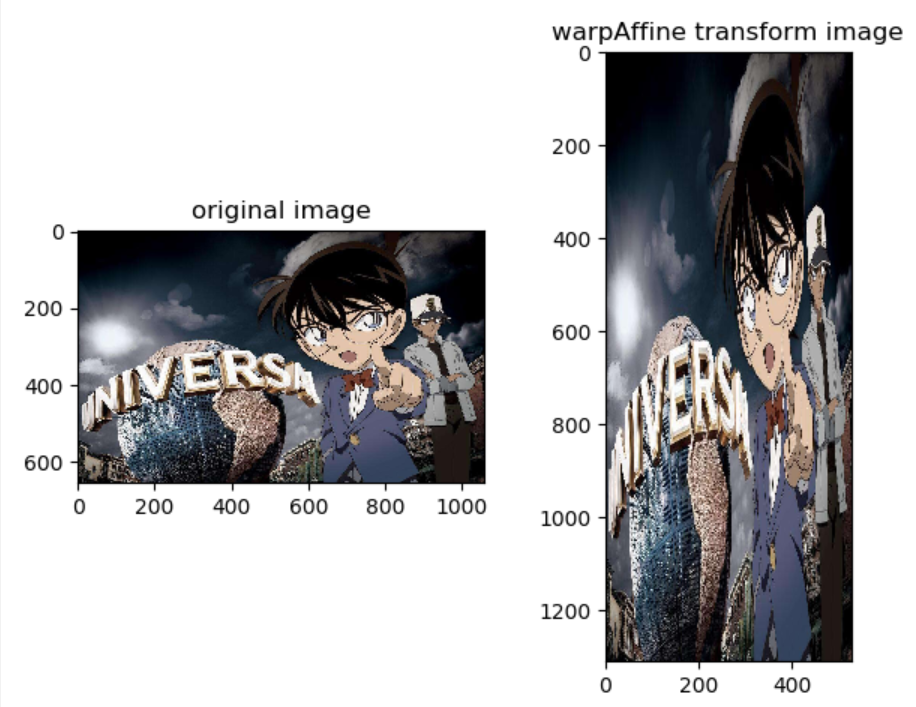这里使用仿射变换实现的图像缩放其实和resize函数的效果是一样的
图像旋转
围绕原点旋转 我们先来看看一个二维平面上的点在围绕原点是如何旋转的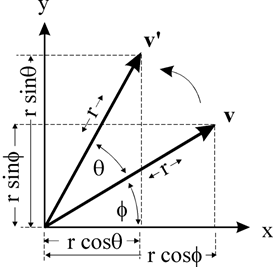上图中点

v

v

在围绕原点旋转

θ

\theta

度之后得到了点

v

′

v'

，我们将坐标点用极坐标的形式来表示可以得到

v

(

r

c

o

s

ϕ

,

r

s

i

n

ϕ

)

v(rcos\phi,rsin\phi)

，所以

v

′

(

r

c

o

s

(

θ

+

ϕ

)

,

r

s

i

n

(

θ

+

ϕ

)

)

v'(rcos(\theta+\phi),rsin(\theta+\phi))

利用正弦和余弦将其展开可得

对

于

v

点

来

说

：

x

=

r

c

o

s

ϕ

y

=

r

s

i

n

ϕ

对

于

v

′

来

说

：

x

′

=

r

c

o

s

(

θ

+

ϕ

)

=

r

c

o

s

θ

∗

c

o

s

ϕ

−

r

s

i

n

θ

∗

s

i

n

ϕ

y

′

=

r

s

i

n

(

θ

+

ϕ

)

=

r

s

i

n

θ

∗

c

o

s

ϕ

+

r

c

o

s

θ

∗

s

i

n

ϕ

然

后

再

将

x

和

y

代

入

上

式

，

可

得

x

′

=

x

∗

c

o

s

θ

−

y

∗

s

i

n

θ

y

′

=

x

∗

s

i

n

θ

+

y

∗

c

o

s

θ

\begin{aligned} 对于v点来说：\\ & x = rcos\phi\\ & y = rsin\phi \\ 对于v'来说：\\ x' &= rcos(\theta+\phi) \\ &=r cos\theta * cos\phi - r sin\theta * sin\phi \\ y' &= rsin(\theta+\phi) \\ &= rsin\theta*cos\phi + rcos\theta * sin\phi \\ 然后再将x和y代入上式，可得 \\ x' &= x * cos\theta-y*sin\theta \\ y' &= x * sin\theta + y * cos\theta \\ \end{aligned}

然后再将上式用矩阵

M

M

表示，可得

[

x

′

y

′

]

=

[

c

o

s

θ

−

s

i

n

θ

0

s

i

n

θ

c

o

s

θ

0

]

[

x

y

1

]

=

M

[

x

y

1

]

\left[ \begin{matrix} x'\\ y'\\ \end{matrix} \right]= \left[ \begin{matrix} cos\theta & -sin\theta & 0\\ sin\theta & cos\theta & 0\\ \end{matrix} \right] \left[ \begin{matrix} x\\ y\\ 1 \end{matrix} \right]= M\left[ \begin{matrix} x\\ y\\ 1 \end{matrix} \right]

特别注意：我们在建立直角坐标系的时候是以左下角为原点建立的，然而对于图像而言是以左上角为原点建立的，所以我们需要对角度

θ

\theta

进行取反，结合三角函数的特性，

M

M

矩阵的表达式如下

M

=

[

c

o

s

θ

s

i

n

θ

0

−

s

i

n

θ

c

o

s

θ

0

]

M= \left[ \begin{matrix} cos\theta & sin\theta & 0\\ -sin\theta & cos\theta & 0\\ \end{matrix} \right]

还需要注意的是这里的角度都是弧度制，所以我们还需要对其进行转换，转换代码如下
#将角度转换为弧度制

将图片围绕原点进行逆时针旋转

θ

\theta

度的代码如下
def rotate_img_original(theta):
#将角度转换为弧度制
#定义围绕原点旋转的变换矩阵
# 读取图像
#定义旋转后图片的宽和高
height,width = img.shape[:2]
# 将图片由BGR转为RGB
img = cv2.cvtColor(img, cv2.COLOR_BGR2RGB)
#围绕原点逆时针旋转\theta度
rotate_img = cv2.warpAffine(img,M,(width,height))
#显示图像
show_compare_img(img,rotate_img)
rotate_img_original(45)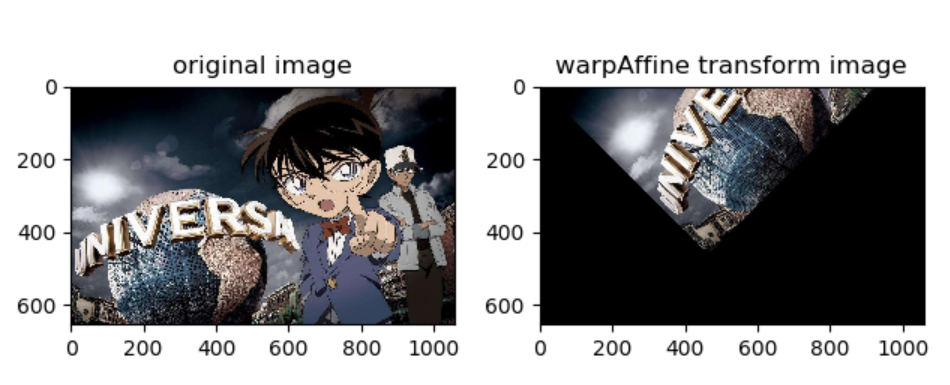围绕任意点旋转 如果我们想围绕任意坐标点旋转呢？其实也并不难，下图的v点在围绕

c

c

点

(

a

,

b

)

(a,b)

旋转90度得到

v

′

v'

。其实我们可以将其等价于，先将

v

v

点平移到

v

1

v_1

点，然后再将

v

1

v_1

点围绕原点旋转90度得到

v

2

v_2

点，最后再将

v

2

v_2

点沿着

v

v

点平移的反方向平移相同长度，最终得到

v

′

v'

。这样我们就将围绕任意坐标点旋转的问题转换成了围绕原点旋转的问题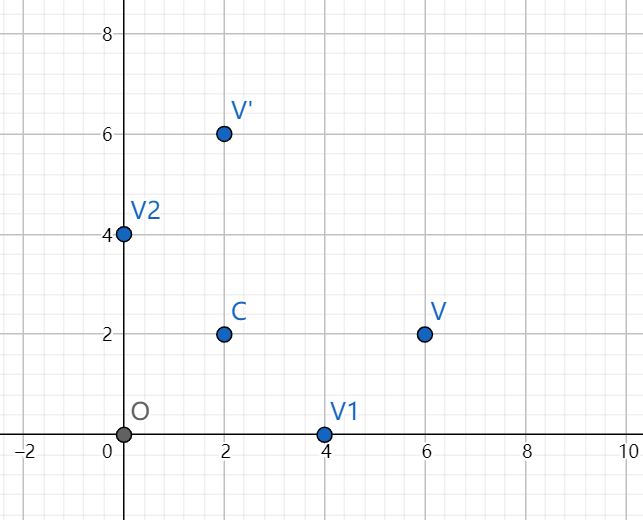我们来回顾一下，围绕原点旋转坐标的变换公式：

x

′

=

x

∗

c

o

s

θ

−

y

∗

s

i

n

θ

y

′

=

x

∗

s

i

n

θ

+

y

∗

c

o

s

θ

\begin{aligned} x' &= x * cos\theta-y*sin\theta \\ y' &= x * sin\theta + y * cos\theta \\ \end{aligned}

在围绕原点旋转变换公式的基础上，我们将其改进为围绕任意点

c

(

a

,

b

)

c(a,b)

旋转，我们现在原来的坐标进行平移，得到变换后的坐标，最后再沿着之前平移的反方向进行平移，就得到围绕任意点旋转的变换公式：

x

′

=

(

x

−

a

)

∗

c

o

s

θ

−

(

y

−

b

)

∗

s

i

n

θ

+

a

y

′

=

(

x

−

a

)

∗

s

i

n

θ

+

(

y

−

b

)

∗

c

o

s

θ

+

b

\begin{aligned} x' &= (x-a) * cos\theta-(y-b)*sin\theta + a \\ y' &= (x-a) * sin\theta + (y-b) * cos\theta + b \\ \end{aligned}

将其展开可得

x

′

=

x

∗

c

o

s

θ

−

y

∗

s

i

n

θ

+

(

1

−

c

o

s

θ

)

∗

a

+

b

∗

s

i

n

θ

y

′

=

x

∗

s

i

n

θ

+

y

∗

c

o

s

θ

+

(

1

−

c

o

s

θ

)

∗

b

−

a

∗

s

i

n

θ

\begin{aligned} x' &= x*cos\theta - y * sin\theta + (1-cos\theta)*a + b * sin\theta\\ y' &= x*sin\theta + y * cos\theta + (1-cos\theta)*b - a*sin\theta\\ \end{aligned}

将上式用矩阵

M

M

表示：

[

x

′

y

′

]

=

[

c

o

s

θ

−

s

i

n

θ

(

1

−

c

o

s

θ

)

∗

a

+

b

∗

s

i

n

θ

s

i

n

θ

c

o

s

θ

(

1

−

c

o

s

θ

)

∗

b

−

a

∗

s

i

n

θ

]

[

x

y

1

]

=

M

[

x

y

1

]

\left[ \begin{matrix} x'\\ y'\\ \end{matrix} \right]= \left[ \begin{matrix} cos\theta & -sin\theta & (1-cos\theta)*a + b * sin\theta\\ sin\theta & cos\theta & (1-cos\theta)*b - a*sin\theta\\ \end{matrix} \right] \left[ \begin{matrix} x\\ y\\ 1 \end{matrix} \right]= M\left[ \begin{matrix} x\\ y\\ 1 \end{matrix} \right]

上式中的c(a,b)表示旋转中心，因为坐标系问题需要对

θ

\theta

进行取反，最终

M

M

矩阵的表达式如下

M

=

[

c

o

s

θ

s

i

n

θ

(

1

−

c

o

s

θ

)

∗

a

−

b

∗

s

i

n

θ

−

s

i

n

θ

c

o

s

θ

(

1

−

c

o

s

θ

)

∗

b

+

a

∗

s

i

n

θ

]

M=\left[ \begin{matrix} cos\theta & sin\theta & (1-cos\theta)*a - b * sin\theta\\ -sin\theta & cos\theta & (1-cos\theta)*b + a*sin\theta\\ \end{matrix} \right]

def rotate_img_point(point_x,point_y,theta,img):
#将角度转换为弧度制
radian_theta = theta / 180 * np.pi
#定义围绕任意点旋转的变换矩阵
# 将图片由BGR转为RGB
img = cv2.cvtColor(img, cv2.COLOR_BGR2RGB)
# 定义旋转后图片的宽和高
height, width = img.shape[:2]
# 围绕原点逆时针旋转\theta度
rotate_img = cv2.warpAffine(img, M, (width, height))
# 显示图像
show_compare_img(img, rotate_img)

height,width = img.shape[:2]
#定义围绕图片的中心旋转
point_x,point_y = int(width/2),int(height/2)
rotate_img_point(point_x,point_y,45,img)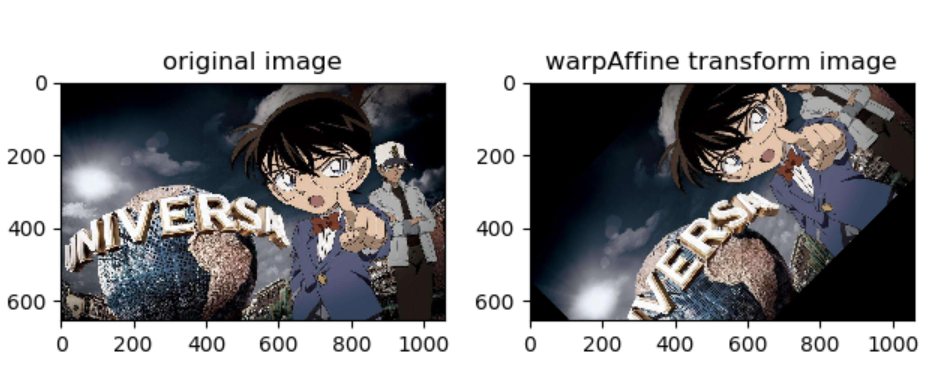细心的同学也许已经发现了，上图中围绕图像中心旋转后的图片部分被裁剪掉了，如果我们想让旋转之后的图片仍然是完整，应该如何修改呢？
def rotate_img_point(point_x,point_y,theta,img,is_completed=False):
#将角度转换为弧度制
radian_theta = theta / 180 * np.pi
#定义围绕任意点旋转的变换矩阵
# 将图片由BGR转为RGB
img = cv2.cvtColor(img, cv2.COLOR_BGR2RGB)
# 定义旋转后图片的宽和高
height, width = img.shape[:2]
#判断旋转之后的图片是否需要保持完整
if is_completed:
#增大旋转之后图片的宽和高,防止被裁剪掉
#增大变换矩阵的平移参数
M[0, 2] += (new_width - width) * 0.5
M[1, 2] += (new_height - height) * 0.5
height = int(np.round(new_height))
width = int(np.round(new_width))
# 围绕原点逆时针旋转\theta度
rotate_img = cv2.warpAffine(img, M, (width, height))
# 显示图像
show_compare_img(img, rotate_img)

height,width = img.shape[:2]
#定义围绕图片的中心旋转
point_x,point_y = int(width/2),int(height/2)
rotate_img_point(point_x,point_y,45,img,True)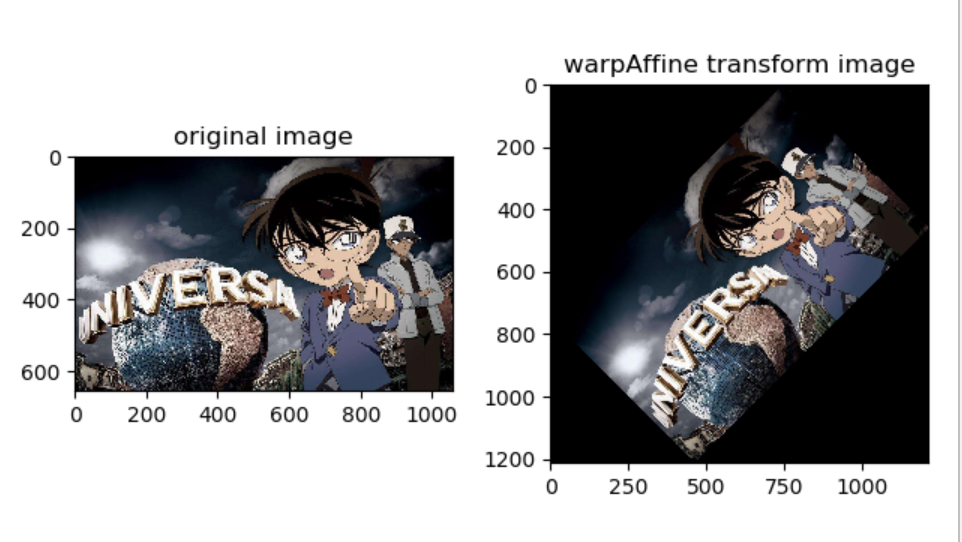展开全文• 仿射变换可以用来平移图像： 进行仿射变换的图像与原图像相比，保持其平直性和平行性。 dst = cv2.warpAffine(src, m, dsize, flags, borderMode, bordervalue) 参数说明： src:原图 dst:结果图像 m:变换矩阵（2*3...
仿射变换可以用来平移图像：
进行仿射变换的图像与原图像相比，保持其平直性和平行性。
dst = cv2.warpAffine(src, m, dsize, flags, borderMode, bordervalue)
参数说明：
src:原图dst:结果图像m:变换矩阵（2*3的矩阵）[[1, 0, dx],[0, 1, dy]]disze:窗口尺寸 (宽（列），高（行）)flags:插值方式borderMode:边类型。bordervalue:边界值，默认0
# 平移 构建平移矩阵
import cv2 as cv
import numpy as np

h, w = img.shape[:2]

# 移动的距离
x = 50
y = 50

# 构建矩阵 float32型 2*3的矩阵
m = np.float32([[1, 0, x], [0, 1, y]])

# 仿射变换进行图像平移 原图像 平移矩阵 dsize尺寸（窗口的尺寸）
dst = cv.warpAffine(img, m, (w, h))

cv.imshow('demo', dst)
cv.waitKey()
cv.destroyAllWindows()复杂的仿射变换：可以进行平移 旋转等，由函数cv2.getAffineTransform()获取转换矩阵。
m = cv2.getAffineTransform(src， src1):
src:原图像的三个点坐标.（左上角，右上角，左下角） src1:结果图像的对应的三个点坐标。 m:转换矩阵
注意：生成三个点的坐标矩阵时用np.float32(一个包括三个点坐标的二维数组)。
# 仿射变换中原图像平行的线，变换后也要平行。转换矩阵由函数获得。

import cv2 as cv
import numpy as np

rows, cols = img.shape[:2]

plt1 = np.float32([[50, 50],[150, 50],[50, 150]])
plt2 = np.float32([[100, 100],[200, 100],[100, 200]])

m = cv.getAffineTransform(plt1, plt2)

dst = cv.warpAffine(img, m, (cols, rows))

cv.imshow('demo', img)
cv.imshow('demo2', dst)

cv.waitKey()
cv.destroyAllWindows()展开全文OpenCV 几何变换 图像平移
• 写文章不易，如果您觉得此... 仿射变换介绍： 请参考：图解图像仿射变换：https://www.cnblogs.com/wojianxin/p/12518393.html 图像仿射变换之图像平移：https://www.cnblogs.com/wojianxin/p/12519498.html 二...数字图像处理 python实现 图像缩放
• 1. 简述仿射变换和透视变换的基本概念，并用实例说明。 仿射变换 用途 旋转 (线性变换)，平移 (向量加)．缩放(线性变换)，错切，反转 方法 仿射变换是一种二维坐标到二维坐标之间的线性变换，它保持了二维图形的...透视变换 代码实现 人工智能
• 常见的2D图像变换从原理上讲主要包括基于2×3矩阵的仿射变换和基于3×3矩阵透视变换。 仿射变换 原理 基本的图像变换就是二维坐标的变换：从一种二维坐标(x,y)到另一种二维坐标(u,v)的线性变换： 如果写成矩阵的...
• 从直观的角度看，仿射变换和透视变换的最大区别是：一个平行四边形，经过仿射变换后依然是平行四边形；而经过透视变换后只是一个四边形（不再平行了）。 仿射变换 仿射变换是把一个二维坐标系转换到另一个二维坐标...
• 仿射变换中，原图中所有的平行线在结果图像中同样平行。为了创建这个矩阵需要从原图像中找到三个点以及他们在输出图像中的位置。然后cv2.getAﬃneTransform 会创建一个 2x3 的矩阵，最后这个矩阵会被传给函数 cv2....
• 1.4 Python图像的坐标变换-仿射变换 文章目录1.4 Python图像的坐标变换-仿射变换1 算法原理2 代码3 效果 1 算法原理 图像的几何变换，也就是矩阵乘法。 通过仿射变换将图片中的每个像素点按照一定的规律映射到新的...python opencv 计算机视觉
• 仿射变换和透视变换引言仿射变换和透视变换概念OpenCV函数封装 引言 我们在拍摄物体时，由于存在倾斜，拍摄图像中的物体难免会出现形变！因此我们需要对此类物体进行矫正处理！ 仿射变换和透视变换概念 其实仿射...
• 仿射变换过程，(x,y)表示原图像中的坐标，(x',y')表示目标图像的坐标 ↑ 二. 仿射变换倾斜算法原理： 原图像大小为h*w X-sharing： 倾斜之x轴逆时针旋转tx度时的图像仿射变换公式 ↑ Y-sharing： 倾斜...图像倾斜 python实现 数字图像处理
• 从直观的角度看，仿射变换和透视变换的最大区别是：一个平行四边形，经过仿射变换后依然是平行四边形；而经过透视变换后只是一个四边形（不再平行了）。 仿射变换 仿射变换是把一个二维坐标系转换到另一个二维坐标...
• 仿射变换 仿射变换是一种二维坐标到二维坐标之间的线性变换，它保持了二维图形的“平直性”（直线经过变换之后依然是直线）和“平行性”（二维图形之间的相对位置关系保持不变，平行线依然是平行线，且直线上点...
• 原理单应性变换（Homography）齐次坐标系单应性变换SVD(奇异值分解）单应性变换算法代码仿射变换（affine）参考： 1.原理 单应性变换（Homography） 齐次坐标系 齐次坐标系(x,y,wx,y,wx,y,w)与常见的三维空间坐标系...
• 一、仿射变换(Affine Transformation)  放射变换（平面变换、二维坐标变换）：是空间直角坐标系的变换，从一个二维坐标变换到另一个二维坐标，仿射变换是一个线性变换，保持了图像的“平行性”和“平直性”，即...图像处理 透视
• 本节以绕图像中心点循环旋转的组合仿射变换和以图像中心点开始与x轴成30°夹角的线段作为依赖轴的循环错切的组合仿射变换为例，详细介绍了二者的OpenCV-Python实现。通过相关案例的介绍，对前面4节介绍的仿射变换...python opencv 编程语言
• 文章目录CV — 数据增强：仿射变换一、前言(一) 仿射变换概念(二) 仿射变换矩阵二、代码实现(一) opencv 函数说明1. warpAffine2. warpPerspective(二) 实现代码 CV — 数据增强：仿射变换 一、前言 (一) 仿射变换...opencv 计算机视觉
• 仿射变换： 透视变换： 原理和C++实现请看我的另外一篇文章： https://blog.csdn.net/RayChiu757374816/article/details/120174397 仿射变换： 原图： 测试代码： import cv2 import numpy as np import ...opencv python
• 图像线性变换是仿射变换的子集，包括图像的旋转、错切、缩放以及几者的组合叠加，线性变换的图像和原图像相比，坐标原点保持不变、直线变换后是直线或原点，平行线变换后还是平行。所有图像线性变换都可以使用图像...图形图像处理 线性变换 矩阵变换
• 一. 前言 1. 最近在做身份证OCR项目中，需要对倾斜扭曲的图像做矫正，透视变换正可以解决这个问题，在这里记录对变换方法的理解。...透视变换和仿射变换在原理上比较相似，而仿射变换更加简单。 1. 定义：...
• import cv2 import numpy as np import matplotlib.pyplot as plt img = cv2.imread('drawing.jpg') ...仿射变换 # 变换前的三个点 pts1 = np.float32([[50, 65], [150, 65], [210, 210]]) # ...opencv 图像处理
• 今天我想来谈谈视图几何的3D空间的三种变换方式，分别是相似变换、仿射变换和影射变换。 提示：以下是本篇文章正文内容，下面案例可供参考 一、相似变换 示例：pandas 是基于NumPy 的一种工具，该工具是为了解决...计算机视觉
• 图像仿射变换共有“旋转”、“平移”、“错切（shear）”、“缩放”、“翻转”5种。本文结合keras-retinanet的实现进行分析。之所以采用keras-retinanet进行分析，是因为该实现较为典型，比较容易理解。 keras-......